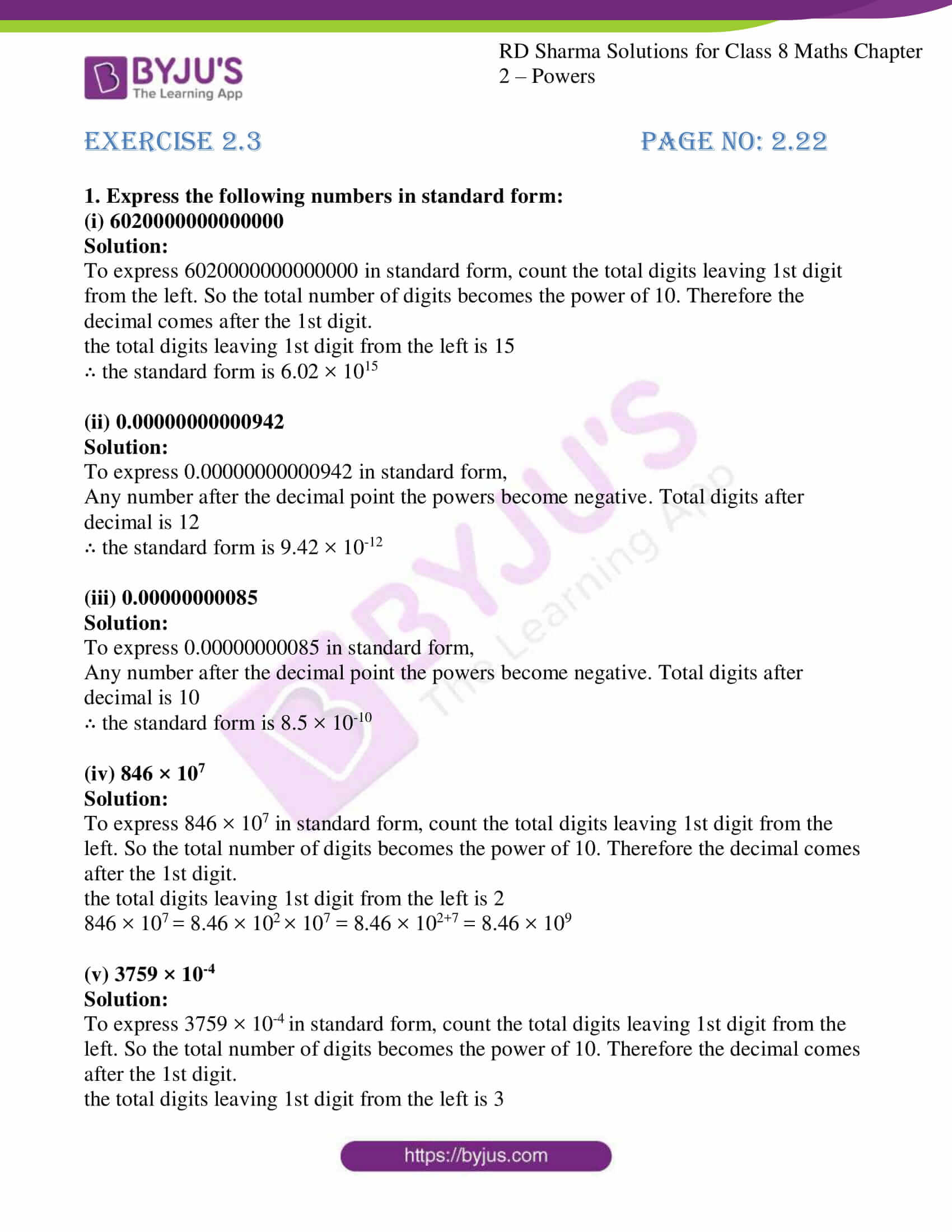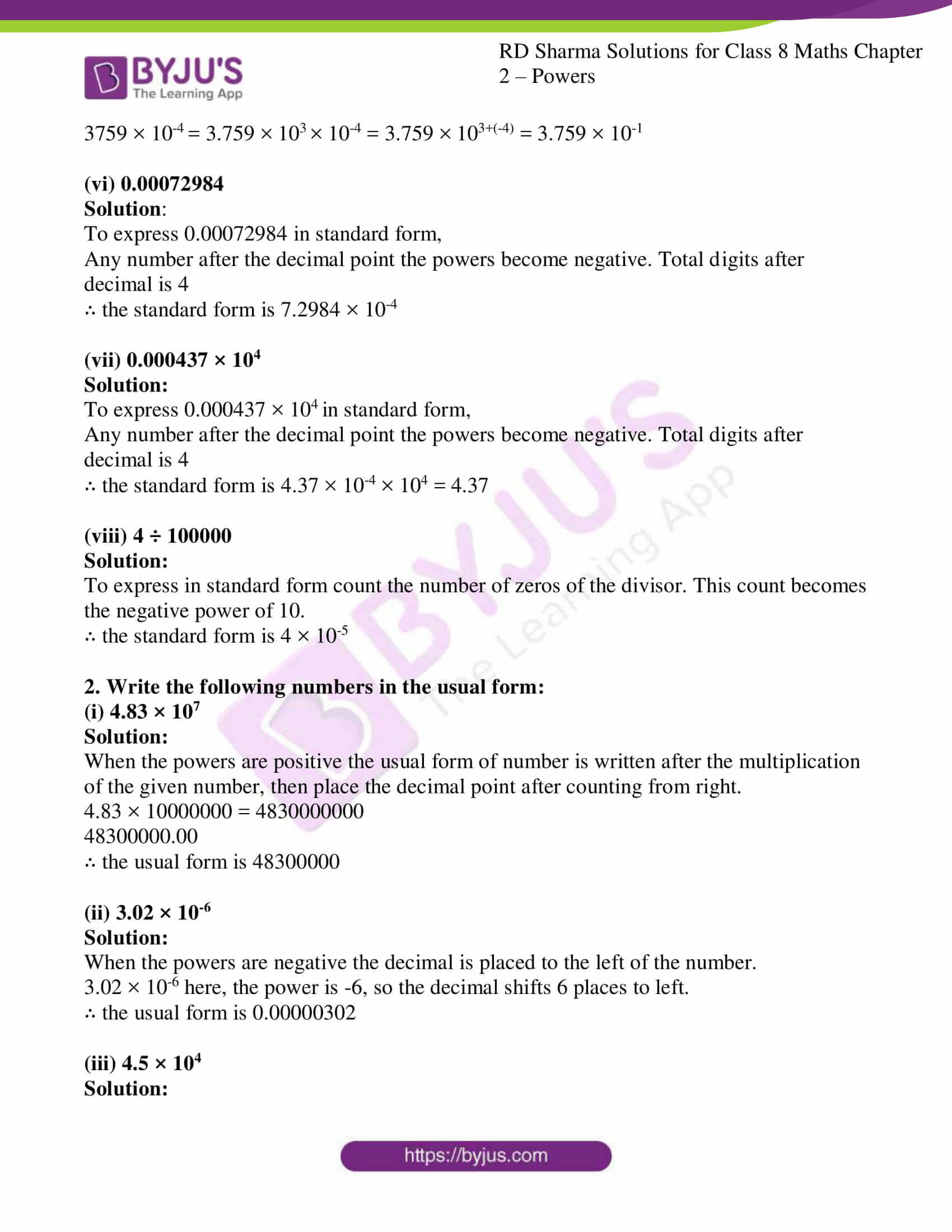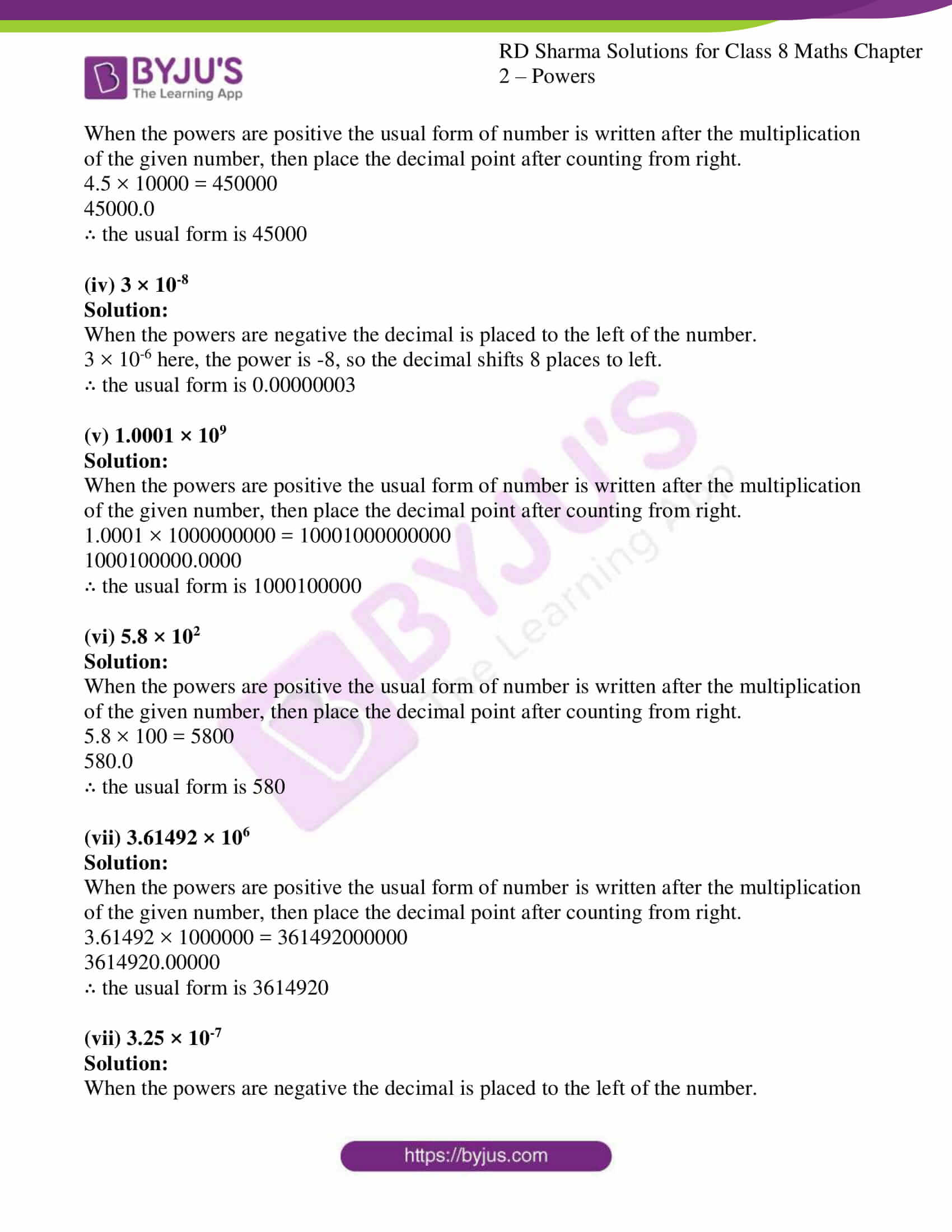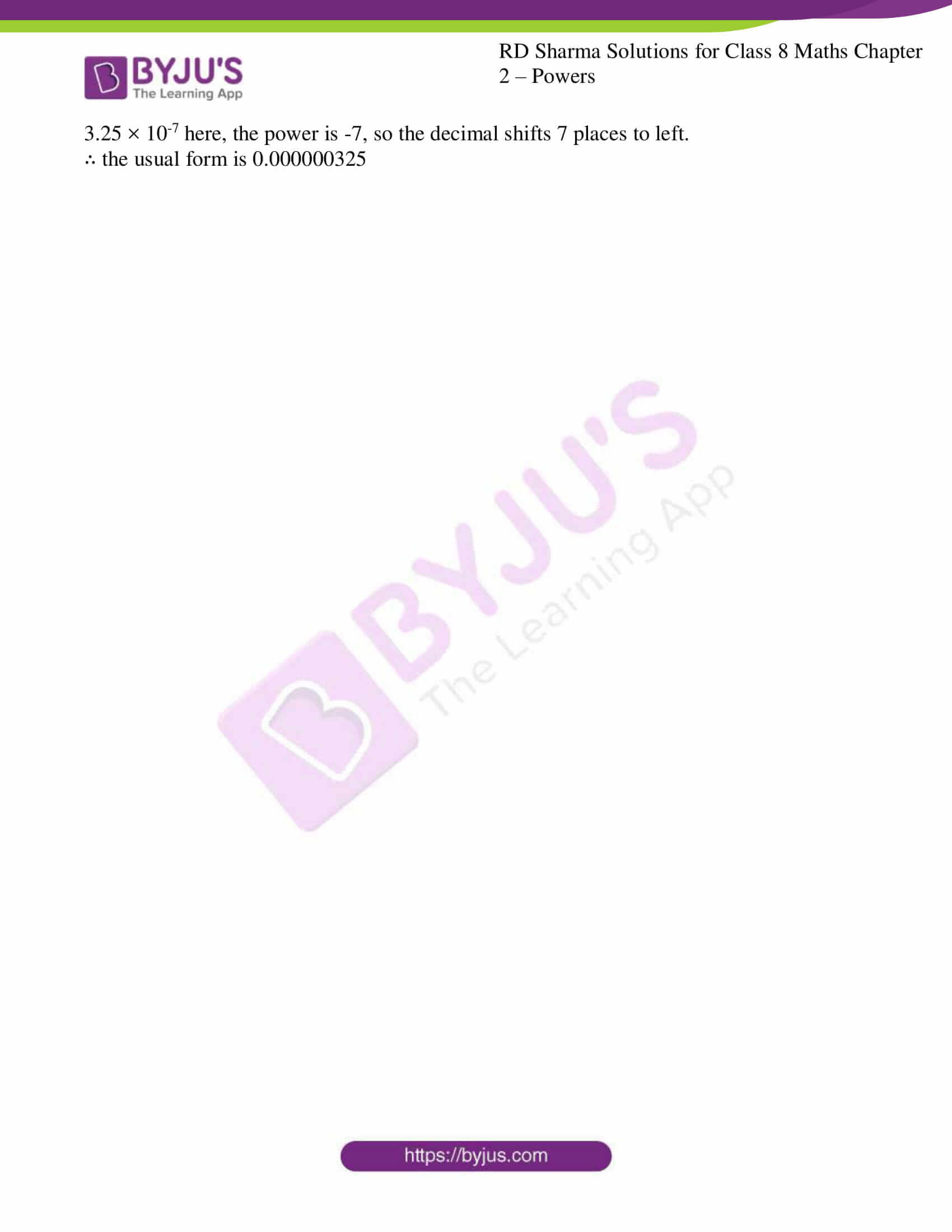# RD Sharma Solutions for Class 8 Maths Chapter 2 - Powers Exercise 2.3

In Exercise 2.3 of RD Sharma Class 8 Maths Chapter 2 Powers, we shall discuss problems based on the use of exponents to express small numbers in standard form. Students can refer and download RD Sharma Solutions Class 8 Maths from the links provided below. Solutions are solved by our experts in order to help students in learning the concepts with ease from the textbook.

## Download PDF of RD Sharma Solutions for Class 8 Maths Exercise 2.3 Chapter 2 Powers### Access Answers to RD Sharma Solutions for Class 8 Maths Exercise 2.3 Chapter 2 Powers

1. Express the following numbers in standard form:

(i) 6020000000000000

Solution:

To express 6020000000000000 in standard form, count the total digits leaving 1st digit from the left. So the total number of digits becomes the power of 10. Therefore the decimal comes after the 1st digit.

the total digits leaving 1st digit from the left is 15

∴ the standard form is 6.02 × 1015

(ii) 0.00000000000942

Solution:

To express 0.00000000000942 in standard form,

Any number after the decimal point the powers become negative. Total digits after decimal is 12

∴ the standard form is 9.42 × 10-12

(iii) 0.00000000085

Solution:

To express 0.00000000085 in standard form,

Any number after the decimal point the powers become negative. Total digits after decimal is 10

∴ the standard form is 8.5 × 10-10

(iv) 846 × 107

Solution:

To express 846 × 107 in standard form, count the total digits leaving 1st digit from the left. So the total number of digits becomes the power of 10. Therefore the decimal comes after the 1st digit.

the total digits leaving 1st digit from the left is 2

846 × 107 = 8.46 × 102 × 107 = 8.46 × 102+7 = 8.46 × 109

(v) 3759 × 10-4

Solution:

To express 3759 × 10-4 in standard form, count the total digits leaving 1st digit from the left. So the total number of digits becomes the power of 10. Therefore the decimal comes after the 1st digit.

the total digits leaving 1st digit from the left is 3

3759 × 10-4 = 3.759 × 103 × 10-4 = 3.759 × 103+(-4) = 3.759 × 10-1

(vi) 0.00072984

Solution:

To express 0.00072984 in standard form,

Any number after the decimal point the powers become negative. Total digits after decimal is 4

∴ the standard form is 7.2984 × 10-4

(vii) 0.000437 × 104

Solution:

To express 0.000437 × 104 in standard form,

Any number after the decimal point the powers become negative. Total digits after decimal is 4

∴ the standard form is 4.37 × 10-4 × 104 = 4.37

(viii) 4 ÷ 100000

Solution:

To express in standard form count the number of zeros of the divisor. This count becomes the negative power of 10.

∴ the standard form is 4 × 10-5

2. Write the following numbers in the usual form:

(i) 4.83 × 107

Solution:

When the powers are positive the usual form of number is written after the multiplication of the given number, then place the decimal point after counting from right.

4.83 × 10000000 = 4830000000

48300000.00

∴ the usual form is 48300000

(ii) 3.02 × 10-6

Solution:

When the powers are negative the decimal is placed to the left of the number.

3.02 × 10-6 here, the power is -6, so the decimal shifts 6 places to left.

∴ the usual form is 0.00000302

(iii) 4.5 × 104

Solution:

When the powers are positive the usual form of number is written after the multiplication of the given number, then place the decimal point after counting from right.

4.5 × 10000 = 450000

45000.0

∴ the usual form is 45000

(iv) 3 × 10-8

Solution:

When the powers are negative the decimal is placed to the left of the number.

3 × 10-6 here, the power is -8, so the decimal shifts 8 places to left.

∴ the usual form is 0.00000003

(v) 1.0001 × 109

Solution:

When the powers are positive the usual form of number is written after the multiplication of the given number, then place the decimal point after counting from right.

1.0001 × 1000000000 = 10001000000000

1000100000.0000

∴ the usual form is 1000100000

(vi) 5.8 × 102

Solution:

When the powers are positive the usual form of number is written after the multiplication of the given number, then place the decimal point after counting from right.

5.8 × 100 = 5800

580.0

∴ the usual form is 580

(vii) 3.61492 × 106

Solution:

When the powers are positive the usual form of number is written after the multiplication of the given number, then place the decimal point after counting from right.

3.61492 × 1000000 = 361492000000

3614920.00000

∴ the usual form is 3614920

(vii) 3.25 × 10-7

Solution:

When the powers are negative the decimal is placed to the left of the number.

3.25 × 10-7 here, the power is -7, so the decimal shifts 7 places to left.

∴ the usual form is 0.000000325

## RD Sharma Solutions for Class 8 Maths Exercise 2.3 Chapter 2 Powers

Class 8 Maths Chapter 2 Powers Exercise 2.3 is based on the problems to see how to write very small numbers in standard form by using certain steps. To facilitate easy learning and understanding of concepts download free RD Sharma Solutions Chapter 2 in PDF format, which provides answers to all the questions. Practising as many times as possible helps students in building time management skills and also boosts the confidence level to achieve high marks.